# Exercise sheet 3 pdfLoading previews...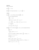second order linear differential equations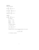second order linear differential equations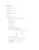second order linear differential equations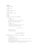second order linear differential equations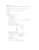second order linear differential equations
5 files in this resource

## Exercise sheet 3 pdf

Exercises and solutions in PDFView Item